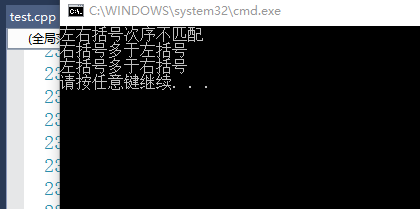# 利用栈检测括号的配对情况

在编程语言中经常使用的括号有（）、[  ]、{  } 这三种，怎么用程序来判断在程序中或者其他情况下这三种括号的配对情况呢?

1、左右括号不匹配--------比如  ( ( abc ) )  12 [ {  ]  }
2、左括号比右括号多-------比如  { [ （（ab13） ]  }
3、右括号比左括号多-------比如 （[ ]）{ 123 } }
4、左右括号匹配成功-------比如  { [ ( 123abc ) ] }

要利用栈来去实现这个过程，那么，首先一定得要明白栈是先进后出或者说是后进先出的特点，明白了这一点，我们再来继续看。
这个包含各种括号的语句其实就相当于一个字符串，那么，我们从第一个字符开始遍历。如果不是括号的话继续往后走，是括号的话就对它进行判断，是左括号还是右括号。左括号的话就将其压入栈中暂时存起来，是右括号的话就让它和栈顶的字符进行比对，在比对之前呢首先得判断一下栈是否为空吧，为空的话很显然右括号就比左括号多了，则为第二种情况，根本就不需要继续比对了。栈不为空的话就看该个右括号与栈顶里的括号是否匹配，如果不匹配的话，就是第一种情况，左右括号次序不匹配。匹配的话就继续往后走。当整个“字符串都遍历完了”，只需对检测栈是否为空，就能将剩下的两种情况区分出来了，栈不为空，说明左括号比右括号多，栈为空，那么，匹配成功！

#include <iostream>
#include <assert.h>
#include <stack>
using namespace std;

bool IsBrackets(char ch)//用于判断是否为括号
{
if (ch == '(' || ch == ')' || ch == '['
|| ch == ']' || ch == '{' || ch == '}')
{
return true;
}
return false;
}

bool MatchBrackets(char* pstr)//检测括号匹配情况
{
assert(NULL != pstr);
stack<char> s;
size_t len = strlen(pstr);
for (size_t i = 0; i < len; i++)
{
//不是括号的话就继续往后走
if (!IsBrackets(pstr[i]))
continue;
else
{
//是左括号就入栈，然后往后走
if (pstr[i] == '(' || pstr[i] == '[' || pstr[i] == '{')
{
s.push(pstr[i]);
}

//是右括号
else
{
//首先判段栈是否为空
//为空则表示右括号多于左括号
if (s.empty())
{
cout << "右括号多于左括号" << endl;
return 0;  //返回0没有什么实际意义，只是为了终止程序
}
//配对成功，栈顶元素出栈
else if (pstr[i] == ')'&&s.top() == '(' ||
pstr[i] == ']'&&s.top() == '[' ||
pstr[i] == '}'&&s.top() == '{')
{
s.pop();
}
else
{
cout << "左右括号次序不匹配" << endl;
return 0;
}
}
}
}
//判断栈是否为空，不为空代表左括号多于右括号
if (!s.empty())
{
cout << "左括号多于右括号" << endl;
return 0;
}
else
return true;
}
 void FunTest()
{
char* str1 = "((abc))[{]}";
char* str2 = "()cd()){[]}";
char* str3 = "[[]()ef{}";
char* str4 = "{[]}[(gg)]()";
MatchBrackets(str1);
MatchBrackets(str2);
MatchBrackets(str3);
MatchBrackets(str4);
}

int main()
{
FunTest();
return 0;
}